Courses

# Test: Constructions- 2

## 25 Questions MCQ Test Mathematics (Maths) Class 10 | Test: Constructions- 2

Description
This mock test of Test: Constructions- 2 for Class 10 helps you for every Class 10 entrance exam. This contains 25 Multiple Choice Questions for Class 10 Test: Constructions- 2 (mcq) to study with solutions a complete question bank. The solved questions answers in this Test: Constructions- 2 quiz give you a good mix of easy questions and tough questions. Class 10 students definitely take this Test: Constructions- 2 exercise for a better result in the exam. You can find other Test: Constructions- 2 extra questions, long questions & short questions for Class 10 on EduRev as well by searching above.
QUESTION: 1

### To divide a line segment AB in the ratio 4 : 7, a ray AX is drawn first such that ∠BAX is an acute angle and then points A1,A2,A3…… are located at equal distances on the ray AX and the point B is joined to:

Solution:

According to the question, point B is joined to A11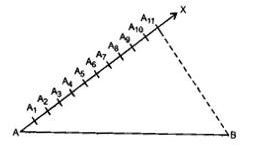QUESTION: 2

### In division of a line segment AB, any ray AX making angle with AB is

Solution:

In division of a line segment AB, any ray AX making angle with AB is an acute angle always.

QUESTION: 3

### Which theorem criterion we are using in giving the just the justification of the division of a line segment by usual method ?

Solution:

Basic Proportionality Theorem (BPT) criterion we are using in giving the just the justification of the division of a line segment by usual method.

QUESTION: 4

To divide a line segment AB in the ratio 5 : 7, first a ray AX is drawn so that ∠BAX is an acute angle and then at equal distances points are marked on the ray AX such that the minimum number of these points is

Solution:

According to the question, the minimum number of those points which are to be marked should be (Numerator + Denominator) i.e., 5 + 7 = 12

QUESTION: 5

To divide a line segment AB in the ration 4 : 7, a ray AX is drawn first such that ∠BAX is an acute angle and then points A1,A2,A3,…. are located at equal distances on the ray AX and the point B is joined to

Solution:

According to the question, the point B is joined to A11.

QUESTION: 6

A line segment drawn perpendicular from the vertex of a triangle to the opposite side is called the

Solution:

In the figure,AA1  =A1A= A2A= A3C = 1 : 4

QUESTION: 7

To divide line segment AB in the ratio A : b ( a, b are positive integers), draw a ray AX so that ∠BAX is an acute angle and then mark points on ray AX at equal distances such that the minimum number of these points is

Solution:

According to the question, the minimum number of those points which are to be marked should be (Numerator + Denominator) i.e., (a + b)

QUESTION: 8

To divide a line segment AB in the ration 5 : 6, draw a ray AX such that ∠BAX is an acute angle, then draw a ray BY parallel to AX and the points A1,A2,A3… and B1,B2,B3.... are located at equal distances on ray AX and BY, respectively. Then, the points joined are

Solution:

According to the question, the points joined are A6 to B5. Because if we have to divide a line segment AB in the ratio m : n, then we draw rays AX and BY and mark the points A1, A2, ……, Am and B1, B2, ……, Bn on rays AX and BY respectively. Then we join the point Am to Bn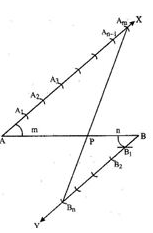QUESTION: 9

To divide a line segment AB in the ration 2 : 3, first a ray AX is drawn so that ∠BAX is an acute angle and then at equal distances, points are marked on the ray AX, such tha the minimum number of these points is

Solution:

According to the question, the minimum number of those points which are to be marked should be (Numerator + Denominator) i.e., 2 + 3 = 5

QUESTION: 10

To divide a line segment AP in the ration 2 : 9, a ray AX is drawn first such that ∠BAX is an acute angle and then points A1,A2,A3... are located of equal distances on the ray AX and the points P is joined to

Solution:

According to the question, the points P is joined to A11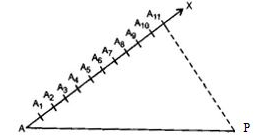QUESTION: 11

To draw a pair of tangents to a circle which are inclined to each other at an angle of 35°, it is required to draw tangents at the end points of those two radii of the circle, the angle between which is :

Solution:

According to the question, the angle between the radii should be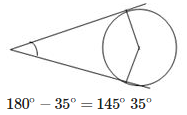QUESTION: 12

To draw a pair of tangents to circle which are inclined to each other at angle of 60°, it is required to draw tangents at end points of those two radii of the circle, the angle between them should be :

Solution:

According to the question, the angle between the radii should be 180°−60°=120°QUESTION: 13

A draw a pair of tangents to a circle which are inclined to each other at an angle of 65°, it is required to draw tangents at the end points of those two radii of the circle, the angle between which is :

Solution:

According to the question, the angle between the radii should be 180°−65°=115°65°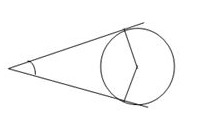QUESTION: 14

To draw a pair tangents to a circle which are inclined to each other at an angle of 70°, it is required to draw tangents at end points of those two radii of the circle, the angle between them should be

Solution:

According to the question, the angle between the radii should be 180°−70° = 110°70°QUESTION: 15

If two tangents are drawn at the end points of two radii of a circle which are inclined at 120° to each other, then the pair of tangents will be inclined to each other at an angle of

Solution:

According to the question, the pair of tangents will be inclined to each other at an angle of 180°−120° = 60°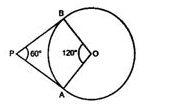QUESTION: 16

To draw a pair of tangents to a circle which are at right angles to each other, it is required to draw tangents at end points of the two radii of the circle, which are inclined at an angle of

Solution:

According to the question, the tangents are inclined at an angle of 180°−90° = 90°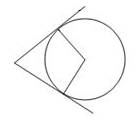QUESTION: 17

To draw a pair of tangents to a circle which are inclined to each other at an angle of 45° it is required to draw tangents at the end points of the two radii of the circle, which are inclined at an angle of

Solution:

According to the question, the angle between radii is 180°−45° = 135°

QUESTION: 18

To draw a pair of tangents to a circle which are inclined to each other at angle x°, it is required to draw tangents at the end points of those two radii of the circle, the angle between which is

Solution:

To draw a pair of tangents to a circle which are inclined to each other at angle x°, it is required to draw tangents at the end points of those two radii of the circle, the angle between them is180°−x°

QUESTION: 19

To draw tangents to each of the circle with radii 3 cm and 2 cm from the centre of the other circle, such that the distance between their centres A and B is 6 cm, a perpendicular bisector of AB is drawn intersecting AB at M. The next step is to draw

Solution:

According to the question, the next step is to draw is a circle with AB as diameter.

QUESTION: 20

To draw tangents to a circle of radius ‘p’ from a point on the concentric circle of radius ‘q’, the first step is to find

Solution:

To draw tangents to a circle of radius ‘p’ from a point on the concentric circle of radius ‘q’, the first step is to find midpoint of q.

QUESTION: 21

To draw a tangent at point B to the circumcircle of an isosceles right ΔABCright angled at B, we need to draw through B

Solution:

To draw a tangent at point B to the circumcircle of an isosceles right ΔABC right angled at B, we need to draw through B in parallel to AC.

QUESTION: 22

A pair of tangents can be constructed to a circle inclined at an angle of :

Solution:

A pair of tangents can be constructed to a circle inclined at an angle of less than 180°. Here it is 175°.

QUESTION: 23

A pair of tangents can be constructed to a circle from an external point, which are inclined each other at an angle θ such that :

Solution:

A pair of tangents can be constructed to a circle from an external point, which are inclined each other at an angle θ such that 0<θ<180°.

QUESTION: 24

Two distinct tangents can be constructed from a point P to a circle of radius 2r situated at a distance:

Solution:

If we have to draw the tangents from any external point of the circle, then the distance of the external point from the centre should be more than the radius of the circle.
Therefore, two distinct tangents can be constructed from a point P to a circle of radius 2r situated at a distance more than 2r from the centre.

QUESTION: 25

Two circles touch each other externally at C and AB is a common tangent to the circles. Then, ∠ACB =

Solution:

Here OA ⊥ AB and O'B ⊥ AB, then ∠OAB = ∠O'BA = 90° And ∠AOO' = ∠BO'O = 90°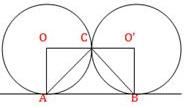∴ ABO'O is a rectangle.
Also, OC = OA, then ∠OCA = ∠OAC= x [Let] ∴ 90° (x + x) = 180°
⇒ x = 45° Again, O'C = O'B, then ∠OC'B = ∠O'BC = y [Let] ∴ 90° (y + y) = 180°
⇒ y =  45°
Now, ∠OCA + ∠ACB + ∠BCO' = 180° [Straight angle]
⇒ 45° +  ∠ACB + 45° = 180°
⇒ ∠ACB = 90°